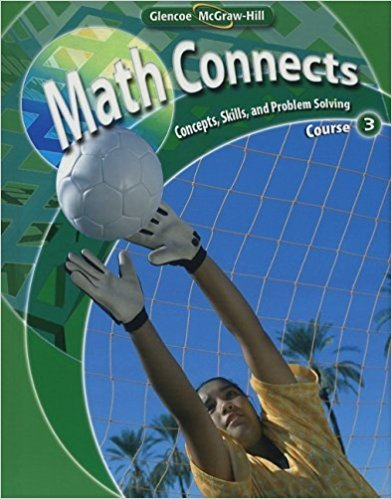×
×

# Solutions for Chapter 9: Math Connects: Concepts, Skills, and Problem Solving Course 3 0th Edition## Full solutions for Math Connects: Concepts, Skills, and Problem Solving Course 3 | 0th Edition

ISBN: 9780078740503Solutions for Chapter 9

Solutions for Chapter 9
4 5 0 294 Reviews
17
5
##### ISBN: 9780078740503

Chapter 9 includes 7 full step-by-step solutions. Math Connects: Concepts, Skills, and Problem Solving Course 3 was written by and is associated to the ISBN: 9780078740503. This textbook survival guide was created for the textbook: Math Connects: Concepts, Skills, and Problem Solving Course 3, edition: 0. Since 7 problems in chapter 9 have been answered, more than 1215 students have viewed full step-by-step solutions from this chapter. This expansive textbook survival guide covers the following chapters and their solutions.

Key Math Terms and definitions covered in this textbook
• Affine transformation

Tv = Av + Vo = linear transformation plus shift.

• Block matrix.

A matrix can be partitioned into matrix blocks, by cuts between rows and/or between columns. Block multiplication ofAB is allowed if the block shapes permit.

• Characteristic equation det(A - AI) = O.

The n roots are the eigenvalues of A.

• Cholesky factorization

A = CTC = (L.J]))(L.J]))T for positive definite A.

• Circulant matrix C.

Constant diagonals wrap around as in cyclic shift S. Every circulant is Col + CIS + ... + Cn_lSn - l . Cx = convolution c * x. Eigenvectors in F.

• Complex conjugate

z = a - ib for any complex number z = a + ib. Then zz = Iz12.

A sequence of steps (end of Chapter 9) to solve positive definite Ax = b by minimizing !x T Ax - x Tb over growing Krylov subspaces.

• Hessenberg matrix H.

Triangular matrix with one extra nonzero adjacent diagonal.

• Hypercube matrix pl.

Row n + 1 counts corners, edges, faces, ... of a cube in Rn.

• Incidence matrix of a directed graph.

The m by n edge-node incidence matrix has a row for each edge (node i to node j), with entries -1 and 1 in columns i and j .

• Network.

A directed graph that has constants Cl, ... , Cm associated with the edges.

• Outer product uv T

= column times row = rank one matrix.

• Particular solution x p.

Any solution to Ax = b; often x p has free variables = o.

• Rayleigh quotient q (x) = X T Ax I x T x for symmetric A: Amin < q (x) < Amax.

Those extremes are reached at the eigenvectors x for Amin(A) and Amax(A).

• Row space C (AT) = all combinations of rows of A.

Column vectors by convention.

• Saddle point of I(x}, ... ,xn ).

A point where the first derivatives of I are zero and the second derivative matrix (a2 II aXi ax j = Hessian matrix) is indefinite.

• Schur complement S, D - C A -} B.

Appears in block elimination on [~ g ].

• Transpose matrix AT.

Entries AL = Ajj. AT is n by In, AT A is square, symmetric, positive semidefinite. The transposes of AB and A-I are BT AT and (AT)-I.

• Vector space V.

Set of vectors such that all combinations cv + d w remain within V. Eight required rules are given in Section 3.1 for scalars c, d and vectors v, w.

• Wavelets Wjk(t).

Stretch and shift the time axis to create Wjk(t) = woo(2j t - k).

×